### Home > MC2 > Chapter 5 > Lesson 5.1.1 > Problem5-8

5-8.
1. Simplify the following expressions. Homework Help ✎

1.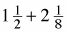2.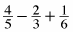3.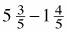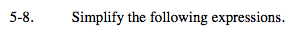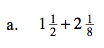First convert these into fractions greater than one.

$3\frac{5}{8}$

Next, find a common denominator and compute the sums.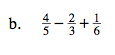Find a common denominator among these three fractions.

Multiply each fraction by a Giant One to convert each fraction to ensure a common denominator.

Compute the sum and differences.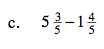See parts (a) and (b).

$3\frac{4}{5}$